## Arf Invariant

A Link invariant which always has the value 0 or 1. A Knot has Arf Invariant 0 if the Knot is pass equivalent'' to the Unknot and 1 if it is pass equivalent to the Trefoil Knot. If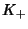,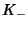, and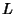are projections which are identical outside the region of the crossing diagram, andandare Knots whileis a 2-component Link with a nonintersecting crossing diagram where the two left and right strands belong to the different Links, then(1)

where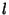is the Linking Number ofand. The Arf invariant can be determined from the Alexander Polynomial or Jones Polynomial for a Knot. For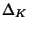the Alexander Polynomial of, the Arf invariant is given by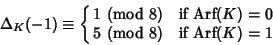(2)

(Jones 1985). For the Jones Polynomial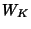of a Knot,(3)

(Jones 1985), where i is the Imaginary Number.

References

Adams, C. C. The Knot Book: An Elementary Introduction to the Mathematical Theory of Knots. New York: W. H. Freeman, pp. 223-231, 1994.

Jones, V. A Polynomial Invariant for Knots via von Neumann Algebras.'' Bull. Amer. Math. Soc. 12, 103-111, 1985.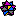Weisstein, E. W. Knots.'' Mathematica notebook Knots.m.